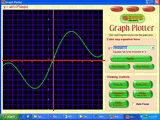## Friday, September 7, 2007

### CBSE Exam India

Preparing for CBSE Exam in India ?

For CBSE - SSLC (10th Standard) & HSC (12th Standard) studentsFile Name: GeniusMakerFree.exe

File Size: 3.5 MB approxThis software is useful for students of CBSE 8th standard to CBSE 12th standard in India.

Alternatively you can visit any of the following sites to download the free software.http://www.goldenkstar.com/ OR http://www.gojocal.org/

Currently this software is extensively used by CBSE students in India, who are preparing for CBSE exams in 2008 and 2009.

This software provides 31 educational software in one pack.

The following are the list of education science software in this pack.

MATHEMATICS SOFTWARE
Graph Plotter The Graph plotting software
Progressions Arithmetic and Geometric progressions
Triangle Solver Triangle solving software
Equation Solver Solving any mathematical equation.
Curve Fitting Polynomial Curve fitting
Analytical Geometry To draw Circles & Lines
Prime Numbers To search and check for Prime numbers
Polynomial Formation Form Polynomial equations from roots
Roots of Polynomial Find roots of polynomial equations
Complex Algebra To do algebra of complex numbers
Matrix Algebra Matrix algebra manipulations
Determinant Finding determinant
Number System Calculator for all number systems
Geometry Solver 2D and 3D Geometry solving software
Polynomial expansion Expansion coefficients for polynomials
Permutation Combination Solving Permutation Combination

PHYSICS SOFTWARE
Unit Converter Converting units
Linear Motion Solving for linear motion
Motion Under Gravity Gravitational vertical motion solver
Projectile Motion Simulate 2D projectile motion of objects
Refraction of Rays Refraction of Rays software
Lens & Mirrors Optics solving software
Doppler Effect Doppler sound effect simulation
Electrical Resistance Resistance circuits
Transverse Waves Visualize Transverse waves
Longitudinal Waves Visualize Longitudinal waves